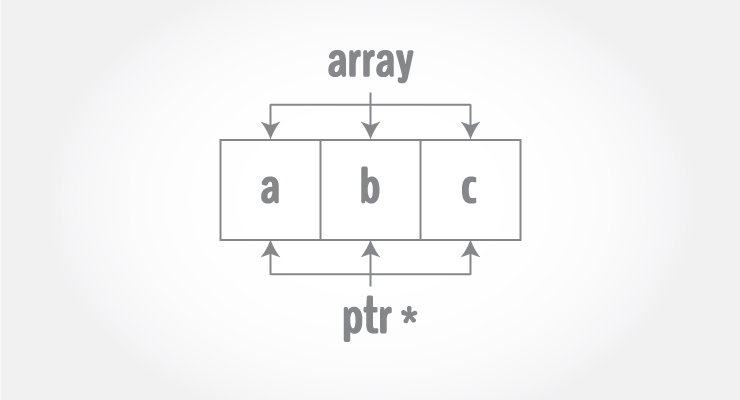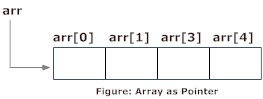C++ Pointers and ArraysPointers are the variables that hold address. Not only can pointers store address of a single variable, it can also store address of cells of an array.

Consider this example:

```int* ptr;
int a;
ptr = &a;  // &a is the address of third element of a.
```Suppose, pointer needs to point to the fourth element of an array, that is, hold address of fourth array element in above case.

Since ptr points to the third element in the above example, `ptr + 1` will point to the fourth element.

You may think, `ptr + 1` gives you the address of next byte to the `ptr`. But it's not correct.

This is because pointer ptr is a pointer to an `int` and size of `int` is fixed for a operating system (size of `int` is 4 byte of 64-bit operating system). Hence, the address between `ptr` and `ptr + 1` differs by 4 bytes.

If pointer ptr was pointer to `char` then, the address between `ptr` and `ptr + 1` would have differed by 1 byte since size of a character is 1 byte.

Example 1: C++ Pointers and Arrays

C++ Program to display address of elements of an array using both array and pointers

``````#include <iostream>
using namespace std;

int main()
{
float arr;
float *ptr;

cout << "Displaying address using arrays: " << endl;
for (int i = 0; i < 5; ++i)
{
cout << "&arr[" << i << "] = " << &arr[i] << endl;
}

// ptr = &arr
ptr = arr;

cout<<"\nDisplaying address using pointers: "<< endl;
for (int i = 0; i < 5; ++i)
{
cout << "ptr + " << i << " = "<< ptr + i << endl;
}

return 0;
}
``````

Output

```Displaying address using arrays:
&arr = 0x7fff5fbff880
&arr = 0x7fff5fbff884
&arr = 0x7fff5fbff888
&arr = 0x7fff5fbff88c
&arr = 0x7fff5fbff890

ptr + 0 = 0x7fff5fbff880
ptr + 1 = 0x7fff5fbff884
ptr + 2 = 0x7fff5fbff888
ptr + 3 = 0x7fff5fbff88c
ptr + 4 = 0x7fff5fbff890
```

In the above program, a different pointer ptr is used for displaying the address of array elements arr.

But, array elements can be accessed using pointer notation by using same array name arr. For example:

```int arr;

&arr is equivalent to arr
&arr is equivalent to arr + 1
&arr is equivalen to arr + 2
```

Example 2: Pointer and Arrays

C++ Program to display address of array elements using pointer notation.

``````#include <iostream>
using namespace std;

int main() {
float arr;

cout<<"Displaying address using pointers notation: "<< endl;
for (int i = 0; i < 5; ++i) {
cout << arr + i <<endl;
}

return 0;
}
``````

Output

```Displaying address using pointers notation:
0x7fff5fbff8a0
0x7fff5fbff8a4
0x7fff5fbff8a8
0x7fff5fbff8ac
0x7fff5fbff8b0```

You know that, pointer ptr holds the address and expression `*ptr` gives the value stored in the address.

Similarly, you can get the value stored in the pointer `ptr + 1` using `*(ptr + 1)`.

Consider this code below:

`int ptr = {3, 4, 5, 5, 3};`
• `&ptr` is equal to `ptr` and `*ptr` is equal to `ptr`
• `&ptr` is equal to `ptr + 1` and `*(ptr + 1)` is equal to `ptr`
• `&ptr` is equal to `ptr + 2` and `*(ptr + 2)` is equal to `ptr`
• `&ptr[i]` is equal to `ptr + i` and `*(ptr + i)` is equal to `ptr[i]`

Example 3: C++ Pointer and Array

C++ Program to insert and display data entered by using pointer notation.

``````#include <iostream>
using namespace std;

int main() {
float arr;

// Inserting data using pointer notation
cout << "Enter 5 numbers: ";
for (int i = 0; i < 5; ++i) {
cin >> *(arr + i) ;
}

// Displaying data using pointer notation
cout << "Displaying data: " << endl;
for (int i = 0; i < 5; ++i) {
cout << *(arr + i) << endl ;
}

return 0;
}
``````

Output

```Enter 5 numbers: 2.5
3.5
4.5
5
2
Displaying data:
2.5
3.5
4.5
5
2```